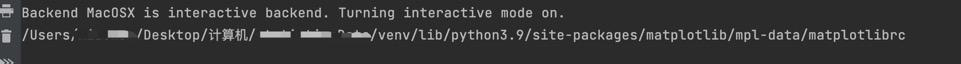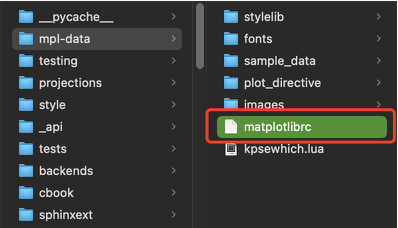# -- coding: utf-8 --

import matplotlib.pyplot as plt

plt.rcParams['font.sans-serif'] = ['Arial Unicode MS'] plt.rcParams['axes.unicode_minus'] = False # 正常顯示負號

X = ['A', 'B', 'C', 'D', 'E', 'F'] #X軸資料 Y = [100, 250, 300, 350, 200, 150] #Y軸資料

plt.style.use('ggplot') #新增網格線 plt.title("柱狀圖示題") #柱狀圖示題 plt.xlabel("X軸名稱") #X軸名稱 plt.ylabel("Y軸名稱") #Y軸名稱

plt.bar(X, Y, 0.4, color="red") #繪製柱狀圖

plt.savefig('柱狀圖1.jpg') # 儲存到本地資料夾，當前路徑下 plt.show() # 顯示柱狀圖

```![柱狀圖.jpg](https://p3-juejin.byteimg.com/tos-cn-i-k3u1fbpfcp/dc2d0e4bb92c4cfb8adf19e2f767315c~tplv-k3u1fbpfcp-zoom-1.image) 這裡有個小問題如果用的是mac電腦中文會顯示亂碼 那應該如何處理呢。 首先匯入matplotlib 在去列印地址``` import matplotlib print(matplotlib.matplotlib_fname()) ```拿到列印地址 command + g 進入 然後編輯檔案matplotlibrc# 準備資料

age20 = manager.age20 / manager.allWorkState age2025 = manager.age2025 / manager.allWorkState age2530 = manager.age2530 / manager.allWorkState age3035 = manager.age3035 / manager.allWorkState age3540 = manager.age3540 / manager.allWorkState age40 = manager.age40 / manager.allWorkState

Yage20 = (round(age20 * 100, 2)) Yage2025 = (round(age2025 * 100, 2)) Yage2530 = (round(age2530 * 100, 2)) Yage3035 = (round(age3035 * 100, 2)) Yage3540 = (round(age3540 * 100, 2)) Yage40 = (round(age40 * 100, 2))

X = ['小於20', '20-25', '25-30', '30-35', '35-40', '40以上'] # X軸資料 Y = [Yage20, Yage2025, Yage2530, Yage3035, Yage3540, Yage40] # Y軸資料

plt.style.use('ggplot') # 新增網格線 plt.title("iOS開發23年3月統計年齡統計") # 柱狀圖示題 plt.xlabel("目前工作年齡 僅做個人開發學習分析") # X軸名稱 plt.ylabel("人數百分比統計") # Y軸名稱

bar = plt.bar(X, Y, 0.4, color="red") # 繪製柱狀圖 plt.bar_label(bar, fmt='%g%%')

plt.savefig('工作年齡.jpg') # 儲存到本地資料夾，當前路徑下 plt.show() # 顯示柱狀圖 ``` 結束了，喜歡我的文章別忘點贊。 另外我還統計了薪資區間 工作的在職離職狀態有需要的可以私信我哈

「其他文章」